Home / Electrical MCQs / AC Circuits MCQs with Answers

# AC Circuits MCQs with Answers

Want create site? Find Free WordPress Themes and plugins.
1. With regards to measuring current and voltage in an AC circuit, modern AC instruments are calibrated to read:

 A. RMS values
 B. peak values
 C. average values
 D. form factor values

2. The current waveform for a purely resistive circuit:

 A. leads the voltage by 90 °
 B. is in phase with the voltage
 C. lags the voltage by 90°
 D. alternately leads and lags the voltage

3. Look at the following figure: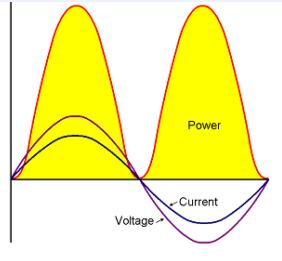Figure shows the voltage, current and power waveshapes for a purely resistive circuit supplied with a sinusoidal AC voltage. The waveshapes show that for a purely resistive circuit, the power curve:

 A. has an average value equal to the area under the voltage wave
 B. has a negative value when both the voltage and current are negative
 C. completes two cycles for each complete cycle of current or voltage
 D. will only have a negative value if the voltage and current are positive

4. The power consumed by a purely resistive AC circuit can be determined using the following formula:
P = Vrms x Irms
In the formula, the symbol ‘P’ stands for the:

 A. peak value of the power
 B. RMS value of the power
 C. maximum value of the power
 D. average value of the power

5. Figure shows a non-inductive resistor:The non-inductive effect is produced by winding:

 A. half in a clockwise direction, and then the other half anticlockwise
 B. coils for magnetic fields inside the inner core from a DC voltage
 C. all the coils in the same direction to produce a self-induced voltage
 D. the resistor with many turns of very fine a high resistance wire

6. A pure resistance of 15 ohms has been connected across an AC power supply that generates a pure sinewave of 84.84 volts peak voltage. The average power consumed by this resistor will be approximately:

 A. 480 W
 B. 240 W
 C. 56 W
 D. 40 W

7. In an inductive AC circuit, the current is continually changing in value and direction, generating an induced EMF that will continually:

 A. assist the change of current flow
 B. assist a change in supply frequency
 C. oppose the change of current flow
 D. oppose the resistance of the circuit conductors

8. Figure 3 shows the waveforms of the voltage and current in a purely inductive AC circuit:Using the voltage phasor as the reference, the current phasor:

 B. is in phase with the voltage
 C. leads and then lags by 90°E
 D. lags by 90°E

9. On AC, the change in current flow gives rise to an induced EMF that opposes the current flow. The effect of this current opposition is called:

 A. inductive reactance
 B. capacitive reactance
 C. inductive resistance
 D. capacitive resistance

10. The inductive reactance in an AC circuit can be calculated from the formula:
XL = 2 π f L
In the formula, the symbol ‘L’ stands for the:

 A. length of the circuit in metres
 B. inductance of the circuit in Henrys
 C. low voltage value of the conductors
 D. frequency of the supply in Hertz

11. A coil has an inductance of 0.04 H. The inductive reactance of the coil at a frequency of 50 Hz will be:

 A. 2 Ω
 B. 0.25 Ω
 C. 12.56 Ω
 D. 6.28 Ω

12. A 230 V 50 Hz supply has been applied to a coil with an inductance of 0.15 H. The current in the circuit will be approximately:

 A. 34.5
 B. A15.3 A
 C. 7.5 A
 D. 4.8 A

13. When inductors are connected in series in an AC circuit, the total inductance can be found using the formula, Ltotal =

 A.B.C.D.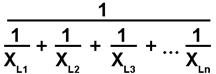14. Two inductors, one with an inductive reactance of 15 Ω, and the second with an inductive reactance of 10 Ω have been connected in series across a 230 V 50 Hz supply. The total inductive reactance will be:

 A. 150 Ω
 B. 25 Ω
 C. 10 Ω
 D. 6 Ω

15. Two inductors, one with an inductive reactance of 12 Ω, and the second with an inductive reactance of 8 Ω have been connected in parallel across a 230 V 50 Hz supply. The total inductive reactance will be:

 A. 20 Ω
 B. 96 Ω
 C. 4.8 Ω
 D. 1.5 Ω

16. Figure shows the voltage, current and power waveshapes for purely inductive circuit:The power waveshape shows that power is:

 A. fed into the inductor continuously
 B. continuously fed into the AC supply
 C. only consumed by the inductor when both V & I are positive
 D. alternately fed into and returned from the inductor

17. A pure inductor with an inductive reactance of 150 W has been connected to a 230 V AC circuit. The average power consumed by this inductor is:

 A. zero
 B. 353 W
 C. 1.53 W
 D. 150 W

18. In a purely capacitive circuit the current:

 A. lags the voltage
 C. is in phase with the voltage
 D. leads and then lags the voltage

19. Figure shows the waveshapes of voltage, current and power for a purely capacitive circuit:In this circuit, the current:

 A. lags the applied voltage by 90°E
 B. leads the applied voltage by 180°E
 C. leads the applied voltage by 90°E
 D. lags the applied voltage by 180°E

20. The capacitive reactance of a capacitor can be determined using the formula:In the formula the symbol ‘f’ stands for the:

 B. speed of the capacitor in m/s
 C. current in the circuit in amperes
 D. frequency of the supply in Hertz

21. A 16 uF capacitor has been connected to a 230 V 50 Hz supply. The capacitive reactance of this capacitor in this circuit will be approximately:

 A. 200 Ω
 B. 14.38 Ω
 C. 80 Ω
 D. 2.00 Ω

22. When two capacitors are connected in series, the total capacitance:

 A. is double the capacitance of any one
 B. is decreased
 C. is increased
 D. remains the same as the largest one

23. When two capacitors are connected in parallel, the total capacitance:

 A. is less than any one
 B. is decreased
 C. is increased
 D. equals the difference between the two

24. An 22 uF capacitor has been connected in series with a 47 uF capacitor. The total capacitance of the combination is approximately:

 A. 69 uF
 B. 15 uF
 C. 47 uF
 D. 22 uF

25. An 16 uF capacitor has been connected in parallel with a 22 uF capacitor. The total capacitance of the combination is approximately:

 A. 38 uF
 B. 22 uF
 C. 16 uF
 D. 9.3 uF

26. Two 10 uF capacitors have been connected in parallel across a 230 V AC supply. The current drawn from the supply will be approximately:

 A. 1.44 A
 B. 0.36 A
 C. 11.5 A
 D. 23.0 A

27. The average power consumed by a purely capacitive circuit:

 A. is maximum when the current is leading
 B. is zero
 C. equals the value of the supply voltage
 D. will be minimum when the current is lagging

28. Figure shows a resistor and an inductor connected in series across an AC supply:In this circuit, the current will:

 A. be in phase with the voltage
 C. lag the voltage
 D. always be a large value

29. Figure shows the phasor diagram for an AC circuit with resistor and capacitor in series: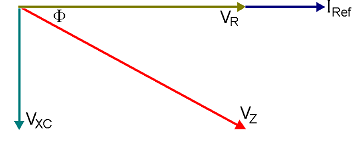The diagram shows that in this type of circuit, the current phasor:

 A. lags the voltage across the capacitor
 B. leads the voltage across the resistor
 C. lags the voltage across the resistor
 D. leads the supply voltage phasor

30. In an AC circuit containing resistance, inductance and capacitance in series, the voltage drop across the inductor will:

 A. be 180°E out of phase with the voltage drop across the capacitance
 B. lead the voltage drop across the capacitance by 90°E
 C. lag the voltage drop across the capacitance by 90°E
 D. be in phase with the voltage drop across the capacitance

31. The following phasor diagram is for a resistor, inductor and capacitor connected in series across an AC supply: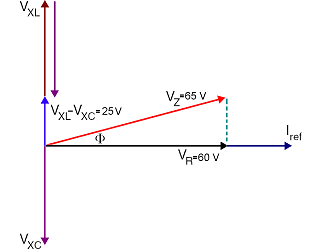The value of the phase angle F is:

 A. 67.4°
 B. 22.6°
 C. 32.5°
 D. 57.5°

32. The following formula can be used to determine the impedance of an AC circuit with resistance, inductance and capacitance in series: Z = √(R2 + (XL – XC)2)
In the formula, the term XL stands for the value of the:

 A. capacitive reactance in Ohms
 B. inductance in Henrys
 C. inductive reactance in Ohms
 D. reactance of the capacitor in microfarads

33. A resistance of 50 Ω has been connected in series with an inductive reactance of 160 Ω and a capacitive reactance of 40 Ω. The impedance of the circuit will be:

 A. 250 Ω
 B. 170 Ω
 C. 190 Ω
 D. 130 Ω

34. In a parallel AC circuit, the voltage is:

 A. common to all the components
 B. equal to the sum of the branch voltages
 C. larger than any branch voltage
 D. equal the phasor sum of the branch currents

35. Figure shows a resistor and inductor connected in parallel across an AC supply:In this circuit, the current through the inductor will:

 B. lag the supply voltage
 C. be in phase with the supply voltage
 D. equal the current through the resistance

36. Figure shows a resistor connected in parallel, with an inductor and resistor in series, across an AC supply.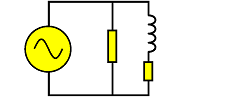In this circuit, the current through the inductor will:

 A. lag the supply voltage by 90°E
 B. lead the supply voltage by 90°E
 C. lead the supply voltage by less than 90°E
 D. lag the supply voltage by less than 90°E

37. Figure 10 shows the phasor diagram for a resistor and capacitor connected in parallel across an AC supply:In this circuit, the current through the capacitor:

 A. leads the supply voltage by 90°E
 B. is in phase with the supply voltage
 C. leads the supply voltage by approximately 30°E
 D. lags the supply voltage by 90°E

38. When drawing the phasor diagram for R, L and C in parallel across an AC supply, the reference phasor is normally the:

 A. current through inductor
 B. supply voltage
 C. current through the capacitor
 D. supply current

39. In an, AC circuit with R, L and C in parallel, the total supply current is:

 A. the arithmetic sum of the branch currents
 B. always equal to zero
 C. the phasor sum of the branch currents
 D. equal to the resistive current minus the inductive current

40. A resistance of 57.5 Ω has been connected to a 230 V 50 Hz supply, in parallel with an inductive reactance of 57.5 Ω and a capacitive reactance of 230 Ω. The total supply current will be:

 A. 9 A
 B. 7 A
 C. 1 A
 D. 5 A

41. An AC circuit with R, L and C in parallel, has the following branch currents.
Resistive branch – 12 A
Inductive branch – 11 A
Capacitive branch – 6 A
The phase angle between the supply voltage and the supply current will be approximately:

 A. 22.6° E
 B. 67.4° E
 C. 56.9° E
 D. 33.1° E

42. When resistance and inductance are combined in one circuit, there will be a value of power consumed that is dependent on the:

 A. capacitive load, in the circuit
 B. resistive load, in the circuit
 C. inductive load, in the circuit
 D. size of the inductive reactance

43. The true power consumed by a single-phase AC circuit can be determined using the formula:

 A. P = V I
 B. P = V I sin f
 C. P = V I cos f
 D. P = V I2

44. In an AC the product of the measured line voltage and line current is called the:

 A. power factor
 B. true power
 C. reactive power
 D. apparent power

45. The reactive power in an AC circuit is sometimes called:

 A. wattless power
 B. apparent power
 C. true power
 D. phase angle power

46. Figure shows the power triangle for an AC circuit:In the diagram, the side marked ‘S’ represents the:

 A. wattless power
 B. apparent power
 C. true power
 D. reactive power

47. In an AC circuit, the power factor is the factor by which the apparent power is multiplied to obtain the:

 A. reactive power
 B. wattless power
 C. true power consumed
 D. phase angle

48. For all electrical power work with sinusoidal waveforms, the power factor is equal to the:

 A. phase angle between the voltage and the current
 B. sine of the phase angle between the voltage and the current
 C. tangent of the phase angle between the voltage and the current
 D. cosine of the phase angle between the voltage and the current

49. Generally, the lower the value of the power factor in an AC circuit, the:

 A. greater will be the current required to supply the same true power
 B. less will be the current required to supply the same true power
 C. less will be the phase angle between the voltage and current
 D. greater will be time taken for the current to pass through

50. One of the major causes of a low power factor is:

 A. transformers running near full load
 C. incandescent lighting
 D. diesel driven alternators

51. The power factor of an AC circuit can be found using the formula, Power factor = :

 A. volt-amperes/watts
 B. volt-amperes x current
 C. watts/volt-amperes
 D. volts x volt-amperes

52. A single phase motor draws 2.175 A from a 230 V supply. A wattmeter in the circuit shows 400 W. The power factor of this circuit is approximately:

 A. 0.57 lagging
 B. 0.6 lagging
 C. 0.76 lagging
 D. 0.8 lagging

53. An inductor draws 11.5 A on 230 V DC and 5.75 A when connected to 230 V AC. The angle of lag between the voltage and current when on AC will be:

 A. 60°
 B. 30°
 C. 45°
 D. 50°

54.
When the switch S1 is closed in figure, the reading on the ammeter will:

 A. increase
 B. decrease
 C. remain the same
 D. be the capacitor current only

55.
When the switch S1 is closed in figure, the power factor of the circuit will:

 A. will be unity
 C. move closer to unity
 D. move toward 0.1 lagging

56. Figure shows the phasor diagram of an electric motor connected to 230 V AC supply: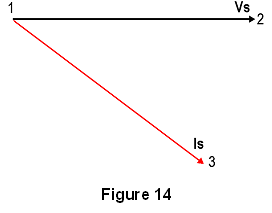If a capacitor is connected in parallel with the motor, the phasor to represent the capacitor current would be drawn:

 A. vertically down from position 1
 B. vertically down from position 3
 C. vertically up from position 2
 D. vertically up from position 1

57. A single-phase 230 V 50 Hz induction motor draws 7.5 A at 0.6 power factor. If a 47 μF capacitor is connected across the line, then the combined line current will be approximately:

 A. 5.2 A
 B. 10.9 A
 C. 4.1 A
 D. 12.5 A

58. The purpose of using capacitors to improve the power factor is to provide a:

 A. lagging current to counteract the lagging current drawn by the load
 C. leading current to counteract the lagging current drawn by the load
 D. lagging current to counteract the leading current drawn by the load

59. When using a power triangle to solve AC circuits the reactive power can be found using the formula:
Q = V I √1 – PF2)
In the formula the symbol PF stands for the:

 B. volt-amps reactive
 C. power factor of the circuit
 D. phase reaction force

60. When an electrical circuit has its power factor corrected to unity, the current is:

 A. lagging the voltage
 C. same value as the voltage
 D. brought into phase with the voltage

61. In an AC circuit, when the capacitive reactance and inductive reactance are exactly equal, the circuit is said to be:

 A. resonant
 B. inductive
 C. capacitive
 D. inductor resistive

62. The major characteristics of the series resonant circuit are a power factor of unity and a:

 A. maximum impedance
 B. minimum impedance
 C. minimum current
 D. maximum capacitive reactance

63. When an inductor and capacitor are connected in parallel and their respective reactances are equal, the reactive currents are:

 A. equal but 90° out of phase with each other
 B. not equal but 180° out of phase with each other
 C. equal but 180° out of phase with each other
 D. not equal but 90° out of phase with each other

64. When the supply frequency to a parallel resonant circuit is varied, the resistance in the circuit is unchanged but the impedance will be:

 A. minimum only at the resonant frequency
 B. maximum at frequencies other than the resonant frequency
 C. maximum only if the resonant frequency is 50 Hz
 D. maximum only at the resonant frequency

65. In an AC circuit at resonance, energy is being transferred back and forth from the:

 A. electromagnetic field of the inductor to the electrostatic field of the capacitor
 B. electrostatic field of the inductor to the electrostatic field of the capacitor
 C. electrostatic field of the inductor to the electromagnetic field of the capacitor
 D. electromagnetic field of the inductor to the electromagnetic field of the capacitor

66. A 10 Ω resistor, 0.25 H inductor and a 40.52 μF capacitor have been connected in series across a variable frequency AC supply. The resonant frequency of the circuit will be:

 A. 40 Hz
 B. 50 Hz
 C. 10 Hz
 D. 40.52 Hz

Did you find apk for android? You can find new Free Android Games and apps.